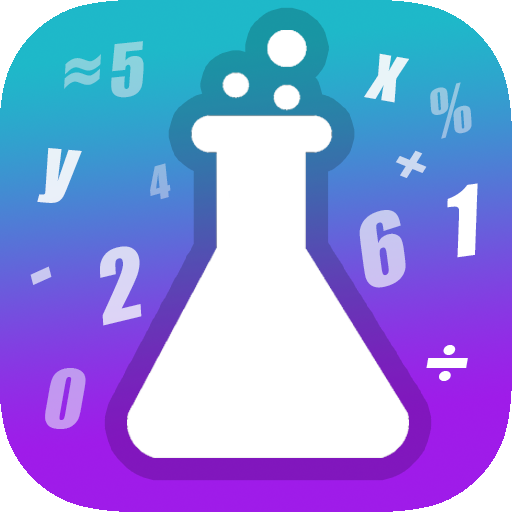# MathLab | Math Puzzles & Riddles

Expand the limits of your intelligence
0 reviews
Do you use MathLab | Math Puzzles & Riddles?
What is MathLab | Math Puzzles & Riddles?
MathLab is a mathematical solving game which tests your IQ with the plenty of math puzzles, riddles and geometrical figures. Gradually, MathLab's math levels get more challenging and make you develop your problem solving skills.